+

# Negative Exponents

Author: Sophia Tutorial
##### Description:

Simplify an expression with negative exponents.

(more)

Sophia’s self-paced online courses are a great way to save time and money as you earn credits eligible for transfer to many different colleges and universities.*

No credit card required

37 Sophia partners guarantee credit transfer.

299 Institutions have accepted or given pre-approval for credit transfer.

* The American Council on Education's College Credit Recommendation Service (ACE Credit®) has evaluated and recommended college credit for 32 of Sophia’s online courses. Many different colleges and universities consider ACE CREDIT recommendations in determining the applicability to their course and degree programs.

Tutorial

what's covered
1. Negative Exponents

# 1. Negative Exponents

There are a few special exponent properties that deal with exponents that are not positive. The first is considered in the following example, which is worded out 2 different ways: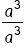Use the quotient rule to subtract exponents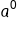Our Solution, but now we consider the problem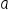the second way:Rewrite exponents as repeated multiplication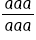Reduce out all the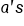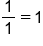Our Solution, when we combine the two solutions, we get: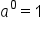Our final result

This final result is an important property known as the zero property of exponents:

formula

Zero Property of ExponentsAny number or expression raised to the zero power will always be 1. This is illustrated in the following example.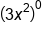Zero power rule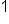Our Solution

Another property we will consider here deals with negative exponents. Again we will solve the following example two ways.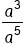Using the quotient rule, subtract exponents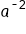Our Solution, but we will also solve this problem another wayRewrite exponents as repeated multiplication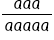Reduce threeout of top and bottom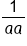Simplify to exponents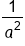Our Solution, putting these solutions together gives: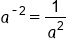Our Final Solution

This example illustrates an important property of exponents. Negative exponents yield the reciprocal of the base. Once we take the reciprocal the exponent is now positive. Also, it is important to note a negative exponent does not mean the expression is negative, only that we need the reciprocal of the base. Following are the properties of negative exponents.

formula

Properties of Negative ExponentsNegative exponents can be combined in several different ways. As a general rule if we think of our expression as a fraction, negative exponents in the numerator must be moved to the denominator, likewise, negative exponents in the denominator need to be moved to the numerator. When the base with exponent moves, the exponent is now positive. This is illustrated in the following example.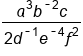Negative exponents on b, d, and e need to flip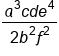Our Solution

As we simplified our fraction we took special care to move the bases that had a negative exponent, but the expression itself did not become negative because of those exponents. Also, it is important to remember that exponents only affect what they are attached to. The 2 in the denominator of the above example does not have an exponent on it, so it does not move with the d.

Simplifying with negative exponents is much the same as simplifying with positive exponents. It is the advice of the author to keep the negative exponents until the end of the problem and then move them around to their correct location (numerator or denominator). As we do this it is important to be very careful of rules for adding, subtracting, and multiplying with negatives. This is illustrated in the following examples: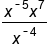Simplify numerator with product rule, adding exponents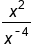Use Quotient rule to subtract exponents, be careful with the negatives!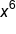Our Solution

EXAMPLE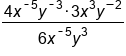Simplify numerator with product rule, adding exponents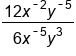Quotient rule to subtract exponents, be careful with negatives!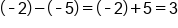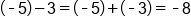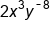Negative exponent needs to move down to denominator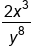Our Solution

EXAMPLE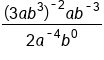In numerator, use power rule with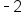, multiplying exponents. In denominator,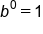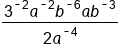In numerator, use product rule to add exponents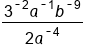Use quotient rule to subtract exponents, be careful with negatives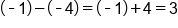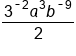Move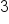and b to denominator because of negative exponents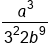Evaluate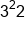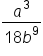Our Solution

hint
In the previous example it is important to point out that when we simplified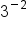we moved the three to the denominator and the exponent became positive. We did not make the number negative! Negative exponents never make the bases negative, they simply mean we have to take the reciprocal of the base.

summary
You can rewrite any negative exponent as positive using one of these two properties. Any base, b, to a negative exponent, -n can be written as 1 over the same base, b, to a positive exponent, n. The exponent goes from negative to positive. We now have our base and exponent in the denominator of the fraction. It's like we have flipped the fraction. Similarly, if you have a fraction, 1 over base, b, to a negative exponent, -n, we can write it as the same base b to the positive exponent n. Again, our exponent goes from negative to positive. Instead of the base and exponent being in the denominator of the fraction, we have it written by itself.
Formulas to Know
Properties of Negative ExponentsZero Property of ExponentsRating